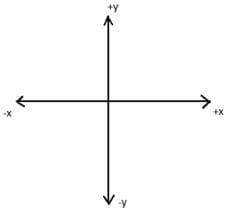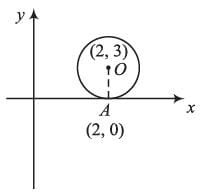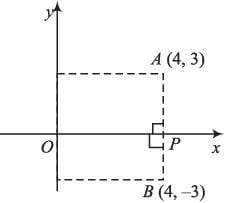Math Olympiad Test: Co-ordinate Geometry- 1

# Math Olympiad Test: Co-ordinate Geometry- 1

Test Description

## 10 Questions MCQ Test Mathematics Olympiad for Class 9 | Math Olympiad Test: Co-ordinate Geometry- 1

Math Olympiad Test: Co-ordinate Geometry- 1 for Class 9 2022 is part of Mathematics Olympiad for Class 9 preparation. The Math Olympiad Test: Co-ordinate Geometry- 1 questions and answers have been prepared according to the Class 9 exam syllabus.The Math Olympiad Test: Co-ordinate Geometry- 1 MCQs are made for Class 9 2022 Exam. Find important definitions, questions, notes, meanings, examples, exercises, MCQs and online tests for Math Olympiad Test: Co-ordinate Geometry- 1 below.
Solutions of Math Olympiad Test: Co-ordinate Geometry- 1 questions in English are available as part of our Mathematics Olympiad for Class 9 for Class 9 & Math Olympiad Test: Co-ordinate Geometry- 1 solutions in Hindi for Mathematics Olympiad for Class 9 course. Download more important topics, notes, lectures and mock test series for Class 9 Exam by signing up for free. Attempt Math Olympiad Test: Co-ordinate Geometry- 1 | 10 questions in 10 minutes | Mock test for Class 9 preparation | Free important questions MCQ to study Mathematics Olympiad for Class 9 for Class 9 Exam | Download free PDF with solutions
 1 Crore+ students have signed up on EduRev. Have you?
Math Olympiad Test: Co-ordinate Geometry- 1 - Question 1

### The coordinate axes (x and y-axis) divide the plane, in how many quadrants?

Detailed Solution for Math Olympiad Test: Co-ordinate Geometry- 1 - Question 1

x-axis and y-axis divide the plane in 4 quadrants.Math Olympiad Test: Co-ordinate Geometry- 1 - Question 2

### The ordinate of any point on x-axis will be

Detailed Solution for Math Olympiad Test: Co-ordinate Geometry- 1 - Question 2

Every point on x-axis is of the form (x, 0)
∴ ordinate of any point on x-axis = 0

Math Olympiad Test: Co-ordinate Geometry- 1 - Question 3

### If the abscissa of a point is negative. The point will lie in

Detailed Solution for Math Olympiad Test: Co-ordinate Geometry- 1 - Question 3

Sign convention for Quadrants are
Ist quadrant → (+, +)
IInd quadrant → (-, +)
IIIrd quadrant → (-, -)
IVth quadrant → (+, -)
∴ Required point will lie in II or III quadrant.

Math Olympiad Test: Co-ordinate Geometry- 1 - Question 4

If a circle is such that x-axis is tangent to it, if the coordinate of centre of circle is (2, 3), then the point of tangency will have ordinate equal to

Detailed Solution for Math Olympiad Test: Co-ordinate Geometry- 1 - Question 4

O is the centre of circle and A is the point of tangency.
∵ Point of tangency lies on x -axis
∴ Ordinate of point = 0Math Olympiad Test: Co-ordinate Geometry- 1 - Question 5

The perpendicular distance of point (-11, -2) from y-axis will be:

Detailed Solution for Math Olympiad Test: Co-ordinate Geometry- 1 - Question 5

Perpendicular distance from y-axis
= |abscissa| = |-11| = 11

Math Olympiad Test: Co-ordinate Geometry- 1 - Question 6

A point on line y = 3x + 2 has equal ordinate and abscissa, then the point will lie in

Detailed Solution for Math Olympiad Test: Co-ordinate Geometry- 1 - Question 6

If  abscissa = ordinate, i.e, x = y then using this relation in equation of line, we have
x = 3x + 2 ⇒ x = -1
∴ Point has coordinates = (-1, -1)
Point has sign convection of (-, -)
∴ Point will lie in 3rd quadrant

Math Olympiad Test: Co-ordinate Geometry- 1 - Question 7

Area of ΔPQR in problem 13 will be:

Detailed Solution for Math Olympiad Test: Co-ordinate Geometry- 1 - Question 7

Area of PQR = 1/2 × PQ × QR
= 1/2 × 4 × 3 = 6 (units)2

Math Olympiad Test: Co-ordinate Geometry- 1 - Question 8

A (2, 3), B (3, 0) and C (14, 13) are vertices of triangle ABC. Then, the centroid of triangle will lie in:

Detailed Solution for Math Olympiad Test: Co-ordinate Geometry- 1 - Question 8

∵ Centroid of any D lies within it, and all the coordinates A, B and C are in Ist quadrant (Positive)
∴ centroid will lie in Ist quadrant.

Math Olympiad Test: Co-ordinate Geometry- 1 - Question 9

The mirror image of point (4, 3) about x-axis will be

Detailed Solution for Math Olympiad Test: Co-ordinate Geometry- 1 - Question 9Point A is 3 units above x-axis.
∴ Its mirror image will be 3 units below x-axis, and the x-coordinate will remain constant
∴ Coordinates of point B ≡ (4, -3)

Math Olympiad Test: Co-ordinate Geometry- 1 - Question 10

A point P on line 2x + 3y = 5, has equal value of both ordinate and abscissa, then the mirror image of point P about y-axis will be:

Detailed Solution for Math Olympiad Test: Co-ordinate Geometry- 1 - Question 10

When ordinate = abscissa, then y = x
∴ 2x + 3x = 5
⇒ 5x = 5
⇒ x = 1
∴ x = y = 1 will be point on line having equal abscissa and ordinate
∴ Point P = (1, 1)
∴ Its image about y-axis will be (-1, 1).

## Mathematics Olympiad for Class 9

1 videos|43 tests
 Use Code STAYHOME200 and get INR 200 additional OFF Use Coupon Code
Information about Math Olympiad Test: Co-ordinate Geometry- 1 Page
In this test you can find the Exam questions for Math Olympiad Test: Co-ordinate Geometry- 1 solved & explained in the simplest way possible. Besides giving Questions and answers for Math Olympiad Test: Co-ordinate Geometry- 1, EduRev gives you an ample number of Online tests for practice

## Mathematics Olympiad for Class 9

1 videos|43 tests